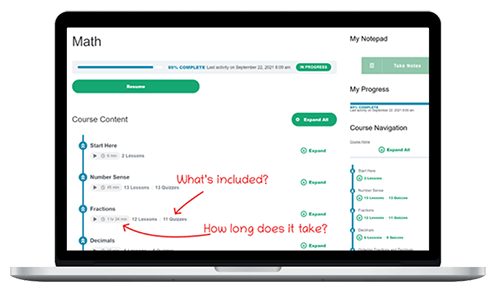# Onsego Math GED Program

The Onsego Math course prepares you to take the math portion of the GED exam successfully. It correlates entirely with the GED Math subtest and includes everything you need to pass the GED® Math test.

## What you’ll learn

• How to apply all mathematical concepts tested on the GED exam
• How to solve problems quickly and accurately
• How to build up confidence in your math skillsThis course includes 16 modules with walkthroughs of math problems ranging in difficulty from easy to more advanced.

At the end of each module, a more extended practice test with full explanations helps you to summarize what you learned.

Every lesson contains a video, text, and a short quiz, and this combination gives students the opportunity to get familiar with the topics in multiple ways. We use a lot of images and explain complex topics in a very graphical, easy-to-understand way.

Every module starts with a diagnostic test that allows you to assess and evaluate your knowledge. If you score high on this test, you can skip the whole module.

Remember that you are allowed to use a calculator during the math test; it must be the TI-30 XS calculator.

## FAQ

How long does it take to prepare for the GED Math test?

The Math course includes 18 learning hours of materials. If you choose to learn math with a calculator, you can shorten the prep time to 11 hours.

So, if you study just 1 hour a day, you will be ready in 2-3 weeks to pass the GED Math test.

What kind of math is on the GED?

You need to be able to solve problems from the following categories:

• Basic Math (percentages, fractions, rates, etc.)
• Geometry (formulas are provided)
• Equations and Inequalities
• Statistics
• Functions
Can I use a calculator?

Yes, for the majority of the questions (40/45), you can use a calculator. However, only one type of calculator is allowed; it has to be the TI-30 XS (scientific) calculator.

How can I learn about using the TI-30 XS calculator?

Onsego has a separate course about using the TI-30 XS calculator. If you choose the My Advantage plan, the TI-30 XS calculator is included. The Math Essentials course doesn’t include the TI-30 XS course. You can read about the TI-30 XS calculator course here.

Can I check how it works?

Sure thing, here is a sample math lesson and a quiz question related to this lesson.

Sample GED Math Lesson

Finding Unit Rates & Unit Prices

Finding Unit Rates

Unit rates compare quantities to 1 unit of the measure. Often, you’ll see the speed of objects traveling expressed in terms of unit rates.

For instance, when we want to describe the speed of a guy riding his bike—and we have the measurement of his distance traveled in $$2$$ hours in miles-we would probably express his speed by giving the distance he traveled in 1 hour.

Now, this is what we call a unit rate. This tells us the distance traveled in 1 hour. So a unit rate’s denominator will be 1, always.

Another example. Consider a car that traveled, in $$5$$ hours$$,300$$ miles. If we want to find the unit rate here, we have to look for the number of miles this car traveled in 1 hour.

$$\frac{300\:miles\,\div\,5}{5\:hours\,\div\,5} = \frac{60\:miles}{1\:hour}[/la tex] We usually write this unit rate as: [latex]60$$ miles per hour.

Finding Unit Prices

Unit prices are unit rates that describe the prices of things. Unit prices describe the prices of single units, always. This helps us to compare prices easily.

Perhaps you have noticed that in groceries, the shelves mark both unit prices and the total prices of products. Well, unit prices make it a lot easier for shoppers when comparing prices of various brands and varying package sizes.

Now, how do we find unit prices?

Here’s an example. Suppose a shopper wants to work with unit prices when comparing a $$3-pack\, of\, tissue$$ that costs $$\4.98$$ to one single box of tissue that costs $$\1.60$$. Now, which offer is the best deal?

Well, then we’ll have to find our unit price of the $$3-pack:\frac{\4.98}{3\,boxes}$$.

Because the given price applies to for $$3$$ boxes, we’ll have to divide here both our denominator and our numerator by $$3$$ if we want to determine the price of $$one$$ box, our unit price. So our unit price here is $$\1.66$$ per box.

So we found that the unit price in our example of the $$3-pack$$ tissue is $$\1.66$$ per single box. When we compare this to a single box a/price, $$\1.60$$, we see that, surprisingly, the $$3-pack$$ tissue box comes at a higher unit price! So buying a single box gives us a better value.

Like unit rates, we often describe unit prices with the expression “per.”

Sometimes, we use a slanted line / to express “per.” Then, the price of our tissue box can be written as $$\frac{\1.60}{box }$$. This is read as $$“\1.60\: per\: box.”$$

Sample Quiz Question

Amazon has a monthly promotion. It says: 3 comic books for 3.9 dollars. What is the unit rate?